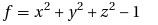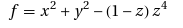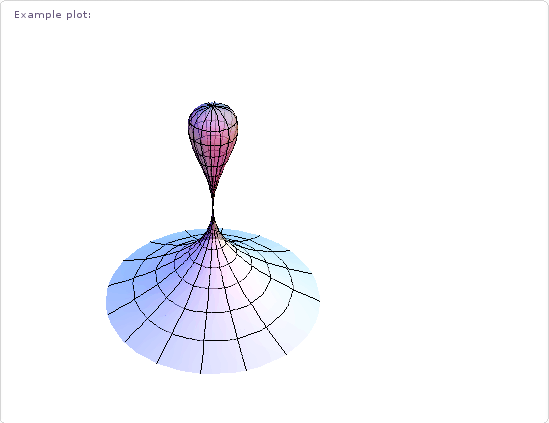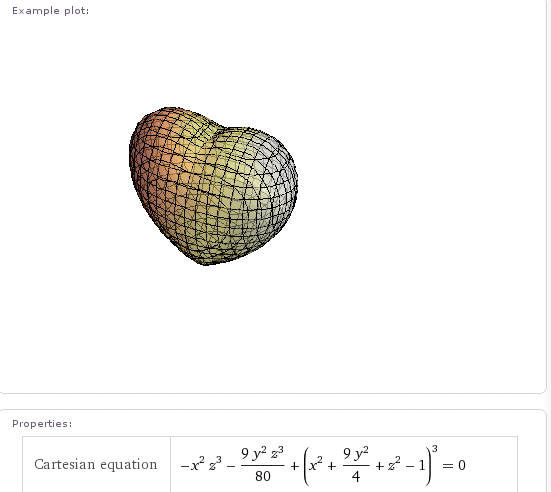## Kiss, love and flirt around with Wolfram Alpha

May 20th, 2009 | Categories: Wolfram Alpha | Tags:

An algebraic surface is a set of solutions to the general equationf(x,y,z)=0 where f is a polynomial in the variables x,y and z.  For example if you set f to the following polynomialthen the set of points x,y and z that satisfyf(x,y,z)=0 forms a sphere of radius 1.  You can get much more interesting surfaces than a sphere though.  For example, setand you’ll get something known as the kiss surface – so called because the bottom section resembles a Hershey’s Kiss. Wolfram Alpha knows all about this surface and if you wolf Kiss Surface you’ll get a whole load of mathematical information about it along with the plot below.One algebraic surface I have looked at in the past is the Heart Surface and it’s pretty obvious to see where it gets its name from.At the time of writing, Wolfram Alpha knows about 129 different, named algebraic surfaces but for some of them it can be a little harder to work out how they got their name. Does anyone know why the one below is called the Flirt Surface for example?Other interesting ones have names like Heaven and Hell,Citrus, Star and Plop!

This functionality is very nice indeed but for it to be really impressive I’d like to see a bit more (I wouldn’t be me if I didn’t want more). For example, it would be great if I could rotate each surface with the mouse – just as I can with 3D images in Mathematica. I’d also like to see more information on each surface such as how it got it’s name or the Mathematica code needed to produce the plot.

What’s your favourite algebraic surface and what extra functionality would you like to see in this area?